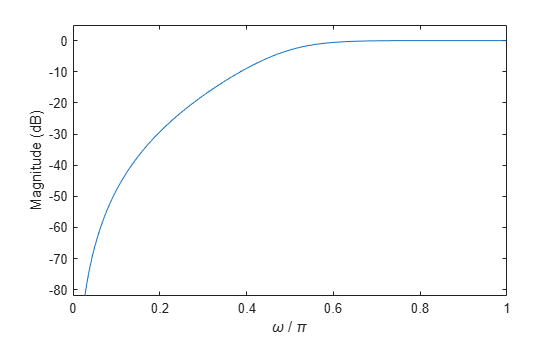Documentation

# mag2db

Convert magnitude to decibels

## Syntax

ydb = mag2db(y)

## Description

example

ydb = mag2db(y) expresses in decibels (dB) the magnitude measurements specified in y. The relationship between magnitude and decibels is ydb = 20 log10(y).

## Examples

collapse all

Design a 3rd-order highpass Butterworth filter having a normalized 3-dB frequency of $0.5\pi$ rad/sample. Compute its frequency response. Express the magnitude response in decibels and plot it.

[b,a] = butter(3,0.5,'high'); [h,w] = freqz(b,a); dB = mag2db(abs(h)); plot(w/pi,dB) xlabel('\omega / \pi') ylabel('Magnitude (dB)') ylim([-82 5])Repeat the computation using fvtool.

fvtool(b,a)## Input Arguments

collapse all

Input array, specified as a scalar, vector, matrix, or N-D array. When y is nonscalar, mag2db is an element-wise operation.

Data Types: single | double

## Output Arguments

collapse all

Magnitude measurements in decibels, returned as a scalar, vector, matrix, or N-D array of the same size as y.